Publicité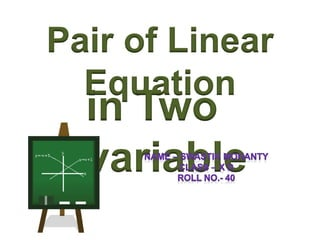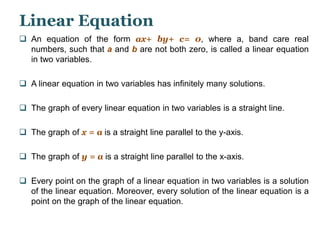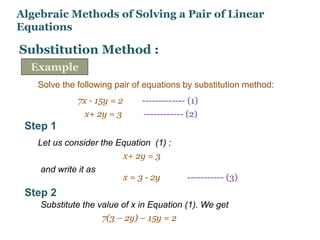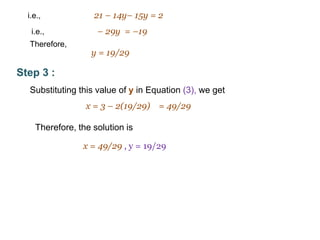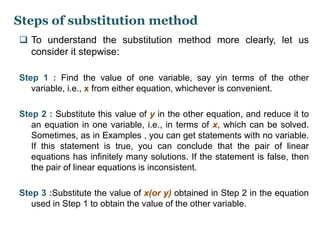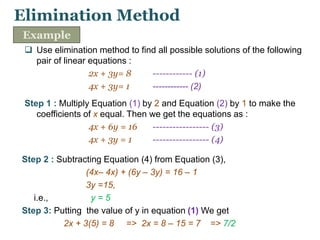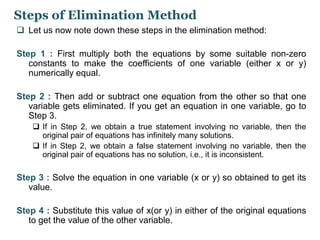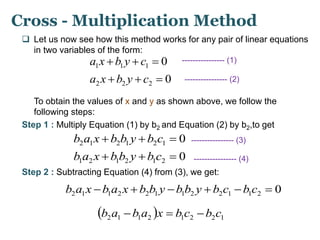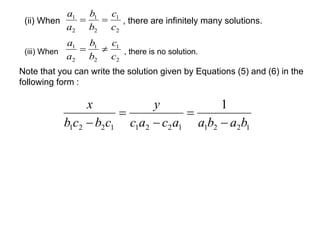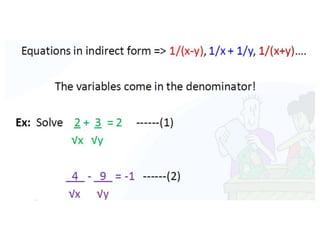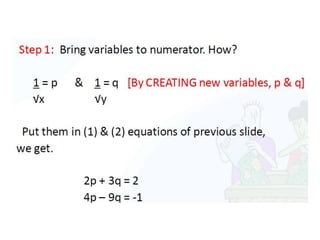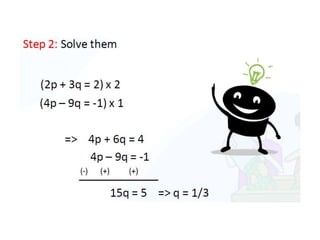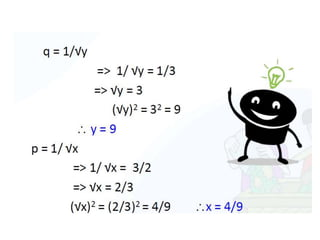1 sur 15
Publicité

### Pair of linear equations in two variables for classX

1. Pair of Linear Equation in Two variable
2. Linear Equation  An equation of the form ax+ by+ c= 0, where a, band care real numbers, such that a and b are not both zero, is called a linear equation in two variables.  A linear equation in two variables has infinitely many solutions.  The graph of every linear equation in two variables is a straight line.  The graph of x = a is a straight line parallel to the y-axis.  The graph of y = a is a straight line parallel to the x-axis.  Every point on the graph of a linear equation in two variables is a solution of the linear equation. Moreover, every solution of the linear equation is a point on the graph of the linear equation.
3. Algebraic Methods of Solving a Pair of Linear Equations Substitution Method : Example Solve the following pair of equations by substitution method: 7x - 15y = 2 ------------- (1) x+ 2y = 3 ------------ (2) Let us consider the Equation (1) : x+ 2y = 3 and write it as x = 3 - 2y ----------- (3) Step 1 Step 2 Substitute the value of x in Equation (1). We get 7(3 – 2y) – 15y = 2
4. i.e., 21 – 14y– 15y = 2 i.e., – 29y = –19 Therefore, y = 19/29 Step 3 : Substituting this value of y in Equation (3), we get x = 3 – 2(19/29) = 49/29 Therefore, the solution is x = 49/29 , y = 19/29
5. Steps of substitution method  To understand the substitution method more clearly, let us consider it stepwise: Step 1 : Find the value of one variable, say yin terms of the other variable, i.e., x from either equation, whichever is convenient. Step 2 : Substitute this value of y in the other equation, and reduce it to an equation in one variable, i.e., in terms of x, which can be solved. Sometimes, as in Examples , you can get statements with no variable. If this statement is true, you can conclude that the pair of linear equations has infinitely many solutions. If the statement is false, then the pair of linear equations is inconsistent. Step 3 :Substitute the value of x(or y) obtained in Step 2 in the equation used in Step 1 to obtain the value of the other variable.
6. Elimination Method Example  Use elimination method to find all possible solutions of the following pair of linear equations : 2x + 3y= 8 ------------ (1) 4x + 3y= 1 ------------ (2) Step 1 : Multiply Equation (1) by 2 and Equation (2) by 1 to make the coefficients of x equal. Then we get the equations as : 4x + 6y = 16 ----------------- (3) 4x + 3y = 1 ----------------- (4) Step 2 : Subtracting Equation (4) from Equation (3), (4x– 4x) + (6y – 3y) = 16 – 1 3y =15, i.e., y = 5 Step 3: Putting the value of y in equation (1) We get 2x + 3(5) = 8 => 2x = 8 – 15 = 7 => 7/2
7. Steps of Elimination Method  Let us now note down these steps in the elimination method: Step 1 : First multiply both the equations by some suitable non-zero constants to make the coefficients of one variable (either x or y) numerically equal. Step 2 : Then add or subtract one equation from the other so that one variable gets eliminated. If you get an equation in one variable, go to Step 3.  If in Step 2, we obtain a true statement involving no variable, then the original pair of equations has infinitely many solutions.  If in Step 2, we obtain a false statement involving no variable, then the original pair of equations has no solution, i.e., it is inconsistent. Step 3 : Solve the equation in one variable (x or y) so obtained to get its value. Step 4 : Substitute this value of x(or y) in either of the original equations to get the value of the other variable.
8. Cross - Multiplication Method  Let us now see how this method works for any pair of linear equations in two variables of the form: a x b y c 0 ---------------- (1) 0    1 1 1 a x b y c    2 2 2 ---------------- (2) To obtain the values of x and y as shown above, we follow the following steps: Step 1 : Multiply Equation (1) by b2 and Equation (2) by b2,to get b a x b b y b c 0 ---------------- (3) 0    2 1 2 1 2 1 b a x b b y b c    1 2 1 2 1 2 ---------------- (4) Step 2 : Subtracting Equation (4) from (3), we get: 0 2 1 1 2 2 1 1 2 2 1 1 2 b a x b a x b b y b b y b c b c    2 1 1 2 1 2 2 1 b a b a x  b c b c
9. It can be observed that the Equations (7) and (2) can both be satisfied only if k c 1 c  kc i e  c 2 1 2 , . ., If c1= kc2 , any solution of Equation (2) will satisfy the Equation (1), and vice versa. k c b a 1 1 1 So, if then there are infinitely many solutions to the pair of linear equations given by (1) and (2). If c1≠ k c2, then any solution of Equation (1) will not satisfy Equation (2) and vice versa. Therefore the pair has no solution. c b a    2 2 2 We can summarize the discussion above for the pair of linear equations given by (1) and (2) as follows: b 1 a 1 (i) When , we get a unique solution. 2 2 b a 
10. c 1 b 1 a 1 (ii) When , there are infinitely many solutions. 2 2 2 c b a   c 1 b 1 a 1 (iii) When , there is no solution. 2 2 2 c b a   Note that you can write the solution given by Equations (5) and (6) in the following form : 1 y  c a c a a b a b x b c b c     1 2 2 1 1 2 2 1 1 2 2 1
Publicité